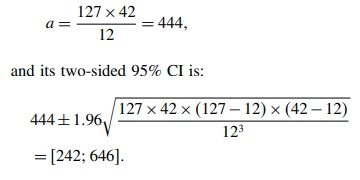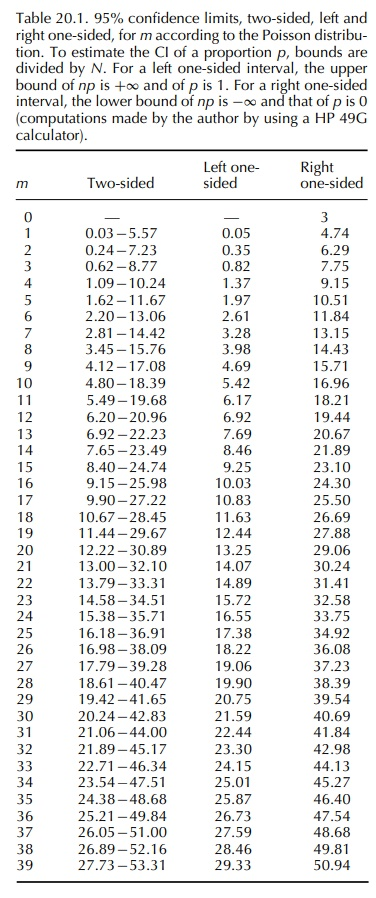# Risk Estimaton From SR

| Home | | Pharmacovigilance |

## Chapter: Pharmacovigilance: Statistical Methods of Evaluating Pharmacovigilance Data

As previously mentioned, the actual number a of cases that have occurred during the surveillance period t could theoretically be estimated by a = k · U,

RISK ESTIMATON FROM SR

### ESTIMATION OF THE NUMERATOR

As previously mentioned, the actual number a of cases that have occurred during the surveillance period t could theoretically be estimated by

a = k · U,

where k is the number of reports during the surveil- lance period and U is the under-reporting coefficient varying from one (exhaustive reporting) to infinite (i.e. the reporting rate is null).

Unfortunately, it is extremely difficult and/or hazardous to estimate the magnitude of this under- reporting, even if in most cases it can be thought to be huge, even for serious cases (Alvarez-Requejo et al., 1998; Eland et al., 1999).

For example, in 1998 a nation-wide prospective study conducted in a representative sample of French public hospitals estimated that 128 768 patients (95% CI: 100 916–156 620) were admitted that year in these hospitals because of an ADR (Pouyanne et al., 2000). This study did not consider other aspects of serious- ness such as death, nor admissions to private hospi- tals. Nevertheless, the obtained figure (128 768) was far larger than the number of serious reactions (about 15 000) reported during the same period to the French pharmacovigilance system still considered as particu- larly efficient.

The capture–recapture approach, when applica- ble, could appear appealing to estimate the total number of cases of a given effect that have occurred in the surveyed population (Jeeger, Schumock and Kong, 1996). This approach derives the size of the source-population from the number of individuals both ‘captured’ by two independent samplings from this population (a more accurate estimate would be obtained by a greater number of samplings, e.g. three or four). To apply this method to pharmacovigi- lance consists in considering two or more independent sources of reports in the same territory. For instance, if k1 and k2 reports have been collected, respectively, during the same period, through two independent sources, for example the regional pharmacovigilance centres network and the concerned manufacturer and if c was the number of duplicates (i.e. cases identified by both sources 1 and 2), then the total number of cases would be

a = k1·k2 / c

If k1 and k2 were large enough (e.g. ≥ 15), the normal approximation can be used to calculate the 1 – α confidence interval (CI) for a:Example: During a one-year surveillance period, 127 cases were reported to the first system and 42 to the second; 12 duplicates were identified. The estimate for the total number of cases isOne can deduce that the actual number of cases has 95 chances in a hundred of being between 242 and 646. The number of cases identified by SR being  127 + 42 12 = 157, the reporting ranges between 24% and 65%.

However, the validity of such an estimate requires that reporting to one system or the other be a truly random and independent phenomenon which could be an unverified assumption. For this reason, the safest way is probably to cease to estimate the actual number of cases and to deal with reporting rates instead of incidence rates!

### ESTIMATION OF THE DENOMINATOR

In some countries, the size N and characteristics of the exposed population and its conditions of expo-sure can be precisely derived from health insurance databases. In this case, except for the poor quality of case collection (i.e. under-reporting), SR approaches the cohort design.

Unfortunately, in most cases, it is necessary to esti-mate these parameters from sales statistics and/or drug prescription on drug utilization panels (Bégaud, Péré and Miremont, 1993). The use of such aggregated data precludes any possibility of considering some individual or sub-group characteristics in the analysis.

The necessary ‘ingredients’ for computation are: the number of exposure units, for example tablets, capsules, injection doses sold in the territory during the relevant period of time, and the average daily dose (ADD) of the considered drug used in this population, the latter being estimated from prescription panels or other sources. By default, the defined daily dose or the recommended daily dose (RDD) can be used as proxy.

Example: 780 000 packages of 20 capsules have been sold in a 1-year period, the used daily dose is 2.1 capsules. This corresponds to the quantity necessary for a cumulative duration of treatment of: (780 000 × 20 2 1 = 2 666 667 days, or 87 719 months. In a more epidemiological parlance, the expo-sure level in the source-population is 87 719 person-months.

As for incidence density calculations, this total probably sums individual exposure periods which are extremely different. Moreover, because of its ecologi-cal character, this approach precludes any risk analysis based on the duration of exposure.

To estimate the number of treatments or the number of subjects treated would require knowing the average duration of a treatment (ADT) with the considered drug. In the previous example, if the ADT was 23 days, the number of treatments for the considered period would be: 2 666 667/23 = 115 942.

However, in the absence of direct information from a health insurance database, the use of measurements made on panels or relatively small samples, both for the average daily dose and duration of treatment, will greatly increase the statistical instability of the esti-mate. In the previous example, if the 95% CIs were [1.6; 2.7] and [16; 31] for the ADD and ADT, respec-tively, then the CI for the number of treatments would range from 31 860 to 104 167. For this reason, it is often preferable to keep person-time estimates for further calculations.

ESTIMATION OF REPORTING RATES

As for incidence rates, the number of cases reported during a given period of time is standardized for the corresponding person-time denominator. For exam-ple, if 18 cases of severe neutropenia have been reported for a cumulative exposure time (estimated from sales statistics) of 87 719 months, the reporting rate is 18/87 719 = 2 05 for 10 000 person-months of exposure. It is sensible to consider that the occur-rence of cases in the exposed population and their reporting, both correspond to a pseudorandom process which can be described by an ad hoc probability model. Given that, in pharmacovigilance, the source popu-lation is generally extremely large and the probabil-ity of occurrence very low, the Poisson distribution is expected to be quite a satisfactory model (Snedecor and Cochran, 1989). In these conditions, the calcula-tion of the 95% two-sided CI for the reporting rate consists of considering the lower and upper limits for the Poisson parameter read in a table such as Table 20.1In the above example, the 95% Poisson CI for the observed number 18 is [10.67; 28.45]. The CI for the reporting rate is thus 10.67 to 28.45 for 87 719 months, that is 1.2 to 3.2 per 10 000 personmonths of exposure. When the number k of reports is large enough, that is 15 or preferably 30, the CI can be calculated by using the normal approximation for a Poisson count (Daly, Bourke and McGilvray, 1991):

CI = k ± Z1−ak

In both cases, this CI defines the set of values which could be observed because of the sampling variation, all parameters remaining identical.